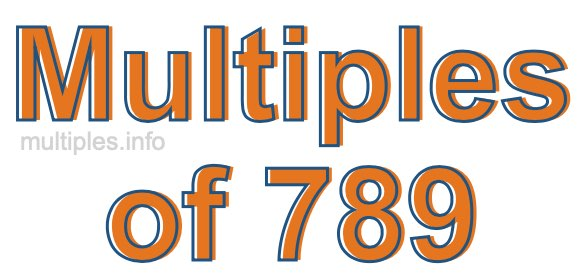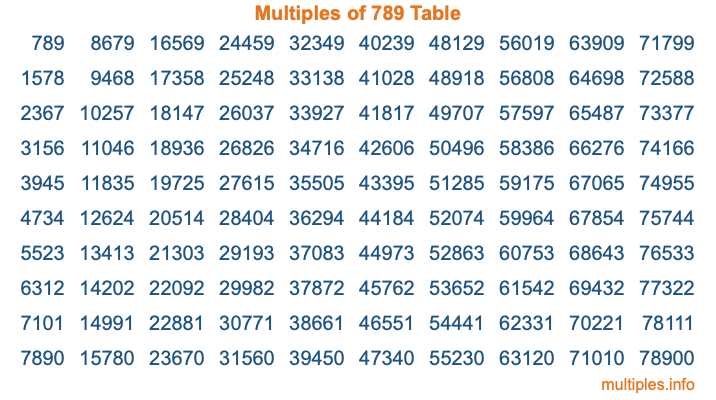Multiples of 789Welcome to the Multiples of 789 page. Here we will first teach you everything you will ever need to know about the multiples of 789, and then give you a study guide summary of everything we taught you to make sure you remember it all. Use this page to look up facts and learn information about the multiples of 789. This page will make you a multiples of seven hundred eighty-nine expert!

Definition of Multiples of 789
Multiples of 789 are all the numbers that when divided by 789 equal an integer. Each of the multiples of 789 are called a multiple. A multiple of 789 is created by multiplying 789 by an integer.

Therefore, to create a list of multiples of 789, you start with 1 multiplied by 789, then 2 multiplied by 789, then 3 multiplied by 789, and so on for as long as you want. Thus, the list of the first five multiples of 789 is 789, 1578, 2367, 3156, and 3945. To see a larger list of multiples of 789, see the printable image of Multiples of 789 further down on this page. We also have a category where you can choose any nth multiple of 789.

Multiples of 789 Checker
The Multiples of 789 Checker below checks to see if any number of your choice is a multiple of 789. In other words, it checks to see if there is any number (integer) that when multiplied by 789 will equal your number. To do that, we divide your number by 789. If the the quotient is an integer, then your number is a multiple of 789.

Is  a multiple of 789?

Least Common Multiple of 789 and ...
A Least Common Multiple (LCM) is the lowest multiple that two or more numbers have in common. This is also called the smallest common multiple or lowest common multiple and is useful to know when you are adding our subtracting fractions. Enter one or more numbers below (789 is already entered) to find the LCM.

Check out our LCM Calculator if you need more details about the Least Common Multiple or if you need the LCM for different numbers for adding and subtraction fractions.

nth Multiple of 789
As we stated above, 789 is the first multiple of 789, 1578 is the second multiple of 789, 2367 is the third multiple of 789, and so on. Enter a number below to find the nth multiple of 789.

th multiple of 789

Multiples of 789 vs Factors of 789
789 is a multiple of 789 and a factor of 789, but that is where the similarities end. All postive multiples of 789 are 789 or greater than 789. All positive factors of 789 are 789 or less than 789.

Below is the beginning list of multiples of 789 and the factors of 789 so you can compare:

Multiples of 789: 789, 1578, 2367, 3156, 3945, etc.

Factors of 789: 1, 3, 263, 789

As you can see, the multiples of 789 are all the numbers that you can divide by 789 to get a whole number. The factors of 789, on the other hand, are all the whole numbers that you can multiply by another whole number to get 789.

It's also interesting to note that if a number (x) is a factor of 789, then 789 will also be a multiple of that number (x).

Multiples of 789 vs Divisors of 789
The divisors of 789 are all the integers that 789 can be divided by evenly. Below is a list of the divisors of 789.

Divisors of 789: 1, 3, 263, 789

The interesting thing to note here is that if you take any multiple of 789 and divide it by a divisor of 789, you will see that the quotient is an integer.

Multiples of 789 Table
Below is an image of the first 100 multiples of 789 in a table. The table is in chronological order, column by column. The first column has the first ten multiples of 789, the second column has the next ten multiples of 789, and so on.The Multiples of 789 Table is also referred to as the 789 Times Table or Times Table of 789. You are welcome to print out our table for your studies.

Negative Multiples of 789
Although not often discussed or needed in math, it is worth mentioning that you can make a list of negative multiples of 789 by multiplying 789 by -1, then by -2, then by -3, and so on, to get the following list of negative multiples of 789:

-789, -1578, -2367, -3156, -3945, etc.

Multiples of 789 Summary
Below is a summary of important Multiples of 789 facts that we have discussed on this page. To retain the knowledge on this page, we recommend that you read through the summary and explain to yourself or a study partner why they hold true.

There are an infinite number of multiples of 789.

A multiple of 789 divided by 789 will equal a whole number.

789 divided by a factor of 789 equals a divisor of 789.

The nth multiple of 789 is n times 789.

The largest factor of 789 is equal to the first positive multiple of 789.

789 is a multiple of every factor of 789.

789 is a multiple of 789.

A multiple of 789 divided by a divisor of 789 equals an integer.

789 divided by a divisor of 789 equals a factor of 789.

Any integer times 789 will equal a multiple of 789.

Multiples of a Number
Here you can get the multiples of another number, all with the same attention to detail as we did for multiples of 789 on this page.

Multiples of
Multiples of 790
Did you find our page about multiples of seven hundred eighty-nine educational? Do you want more knowledge? Check out the multiples of the next number on our list!